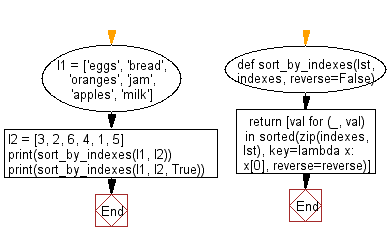﻿ Python: Sort one list based on another list containing the desired indexes - w3resource# Python: Sort one list based on another list containing the desired indexes

## Python List: Exercise - 218 with Solution

Write a Python program to sort one list based on another list containing the desired indexes.

• Use zip() and sorted() to combine and sort the two lists, based on the values of indexes.
• Use a list comprehension to get the first element of each pair from the result
• Use the reverse parameter in sorted() to sort the dictionary in reverse order, based on the third argument

Sample Solution:

Python Code:

``````def sort_by_indexes(lst, indexes, reverse=False):
return [val for (_, val) in sorted(zip(indexes, lst), key=lambda x: \
x, reverse=reverse)]

l1 = ['eggs', 'bread', 'oranges', 'jam', 'apples', 'milk']
l2 = [3, 2, 6, 4, 1, 5]
print(sort_by_indexes(l1, l2))
print(sort_by_indexes(l1, l2, True))
```
```

Sample Output:

```['apples', 'bread', 'eggs', 'jam', 'milk', 'oranges']
['oranges', 'milk', 'jam', 'eggs', 'bread', 'apples']
```

Flowchart:## Visualize Python code execution:

The following tool visualize what the computer is doing step-by-step as it executes the said program:

Python Code Editor:

Have another way to solve this solution? Contribute your code (and comments) through Disqus.

What is the difficulty level of this exercise?

Test your Python skills with w3resource's quiz

﻿

## Python: Tips of the Day

```print(2_000_000)
```2000000